# A Comprehensive Formula for Calculating the Infusion Rate of Medications Diluted with Infusion Solution

1 Inspection Unit, Police Hospital, Kerman, Iran.
2 School of Nursing and Midwifery, Bam University of Medical Sciences, Bam, Iran.

#### Article Metrics

0
##### Total Statistics:

Full-Text HTML Views: 1496
Abstract HTML Views: 73
##### Unique Statistics:

Full-Text HTML Views: 861
Abstract HTML Views: 61open-access license: This is an open access article distributed under the terms of the Creative Commons Attribution 4.0 International Public License (CC-BY 4.0), a copy of which is available at: (https://creativecommons.org/licenses/by/4.0/legalcode). This license permits unrestricted use, distribution, and reproduction in any medium, provided the original author and source are credited.

* Address correspondence to this author at School of Nursing and Midwifery, Bam University of Medical Sciences, Bam, Iran; Tel: 00989132425700;

## DEAR EDITOR,

Drug administration is an integral part of the nurse’s role , and drug calculations are one of the most important nursing care measures . There are various calculating formulas for different drug orders and the multiplicity of these methods has led to the difficulty of various calculations  to the extent that one of the most common nursing errors is mistakes in the calculation of drug dosage especially, the infusion rate of drug solutions . A single formula that can comprise all different aspects of the physician's order, drug dose, and type of infusion set used and ultimately can express infusion rate in terms of drops per minute (gtt/min) and milliliter per hour (ml/hr) can partly reduce this problem. Mistakes in calculating the infusion rate are more important in Iran because of the limited financial resources for supplying the infusion pumps and other automatic injecting devices that have the ability of drug calculation and infusion rate. In this research, researchers tried to innovate a simple, uniform and applicable formula that can be used for a wide range of infusions.

Two of the valid and widely used formulas and their limitations and disadvantages are:

A)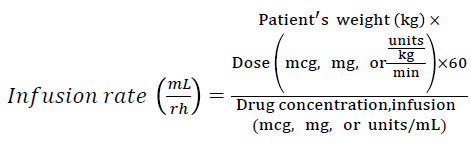The most important limitations include:

(1) It is not able to calculate the infusion rate in gtt/min (because the type of infusion set is not included).

(2) The concentration of the drug should be available. Although the volume of drug solution and the amount of he drug (diluted) are usually present, the calculation of the concentration will prolong the process and the probability of errors increases.

(3) The dose of the drug should be in mcg, mg or a specified unit, while the drugs may be used in different units, such as gram.

(4) The units of drug dose and concentration should be identical, while this formula does not have the ability to make them identical.

B)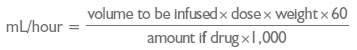The most important limitations include:

(1) It is not able to calculate the infusion rate in gtt/min (because the type of infusion set is not included).

(2) The number 1000 is used for converting mg to mcg. Whereas, if the drug unit is in gram and the order is in mcg, then this formula is not applicable.

The benefits and advantages of our formula:

(1) The infusion rate is calculated in gtt/min, while having the ability to calculate it in mL/hr too (Tips for using this formula No 5).

(2) There is no need to calculate drug concentration.

(3) It is applicable for any type of drug unit.

(4) It is able to make different dose units and drug units identical.

(5) Unlike the two previous formulas, the type of infusion set is included.

The method of obtaining the formula is as follows:

As we know, the infusion rate (when the infusion time is known) is obtained from the following method: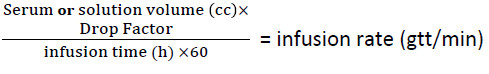On the other hand, if we do not have the total infusion time, we can get it from the following formula using the total dosage of the drug and the physician's order: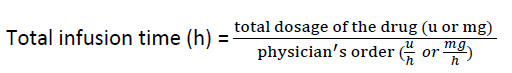By replacing the second formula in the first, considering and applying other conditions (Drug orders based on the patient's weight, the difference of the drug unit with the physician's order, and finally the request for the administration of the infusion of the drug in terms of hours or minutes), the formula for calculating the infusion rate will be as follows: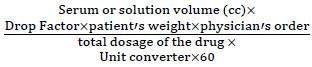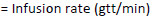## Tips for using this formula:

(1) Regarding the use of this formula, the volume of the serum (or solution) should be in mL, and the physician's order and the total dose of the drug inside the serum (or solution) should be available.

(2) All physician's orders expressed as dose/time, or dose/weight /time, can be calculated and executed using this formula.

(3) The drug dosage can have any unit, but since the unit converter in the denominator is to equalize the unit of the drug dosage with the physician's order, it is only used when the drug unit in solution is not the same as the unit of the physician's order. For example, the drug is in mg, but the physician's order is in mcg (mcg/min, mcg/kg/min, etc.), in which case, the unit converter is 1000 (mcg = 1000mg). So if the dosage of the drug inside the serum (or solution) is the same as the unit of the physician's order (both mg, U, etc.), we will not use the unit converter in the denominator of the fraction.

(4) The drop factor is used in the numerator of the fraction to convert the serum (or solution) volume (ml) to the drop (gtt). In microset, this number is 60 (60 drops = 1 ml) and in the infusion set, it is 10, 15 or 20 (according to the infusion set type).

(5) To use the syringe pump for infusion, you must calculate the infusion rate as milliliter per hour (ml/hr). Also, in some infusion pumps, even using regular infusion set, it is necessary to set the infusion rate as ml/hr. On the other hand, we know that the infusion rate is in microset in gtt/min = ml/hr (1cc =60 gtts and 1h=60min). Therefore, through the microset formula (i.e, taking the number 60 as the drop factor) and taking into account, the solution volume (according to the volume of the syringe, serum, etc.) we reach the answer in gtt /min and set the same number as ml/hr on the infusion pump.

(6) The patient's weight in the numerator of the fraction should be used only for drugs prescribed by the weight (Example: mcg/kg/min). Note that the unit of the physician's order must be the same as the unit of the patient's weight (kg, lb…).

(7) Since the number 60 in the denominator is to convert hr to min, we only use it when the physician's order is expressed in terms of hours (mg/hr, u/hr, mcg/kg/hr, etc.). So, if the physician's order is in minutes (mg/min, mcg, mcg/kg/min, etc.), we will not use the number 60 in the denominator.

(8) Consider that if the dose of the drug in the solution changes (half, twice, etc.), we will use the same modified dose. For example, if 4 ampules of 100mg Lidocaine are poured in the serum (400mg), we use the number 400 in the denominator.

(9) It is recommended that we use this formula for infusions in which the total infusion time is not determined.

### Applicable Example

Q: We want to infuse Midazolam according to the physician's order as 30mcg/kg/hr for a patient weighing 70kg. If we pour three 5mg ampules of this drug in a 20 ml syringe and dilute it to the volume of 20 ml, calculate the infusion rate:

A: As already mentioned, the infusion rate in the syringe pumps is in ml/hr, whereas the final infusion rate of the above formula is in gtt/min. But it has already been determined that in microsets, the infusion rate in gtt/min would be equal to ml/hr. Therefore, the drop factor here is 60.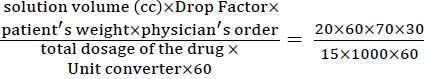=2.8 gtt/min =2.8ml/hr

(1) As the drug is prescribed by weight, then the patient's weight is used in the numerator.

(2) Since the dosage is in mg, but the physician's order is in mcg, the number 1000 is used in the denominator as the unit converter (mcg = mg × 1000).

(3) Since the physician's order is hour (mcg/kg/hr), the number 60 is used in the denominator to convert the unit into minutes.

(4) Because three 5mg ampules are used, the total dosage of the drug in the syringe is considered 15mg

None.

### CONFLICT OF INTEREST

The authors declare no conflict of interest, financial or otherwise.

Declared none.

## REFERENCES

  Bernius M, Thibodeau B, Jones A, Clothier B, Witting M. Prevention of pediatric drug calculation errors by prehospital care providers. Prehosp Emerg Care 2008; 12(4): 486-94.  Khoshab H, Nouhi E, Tirgari B, Ahmadi F. A survey on teamwork status in caring for patients with heart failure: A cross-sectional study. J Interprof Care 2018; 1-7.  Toney-Butler TJ, Wilcox L. Dose Calculation 2018.  Wright K. Student nurses need more than maths to improve their drug calculating skills. Nurse Educ Today 2007; 27(4): 278-85.  Ansel HC. Pharmaceutical calculations 2012.  Lapham R. Drug Calculations for Nurses: A step-by-step approach 2015.